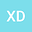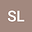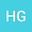LT-SCM-SNI method for solving definite solution problems of three-interval composite nonlinear partial differential equations and its application
•••• +3Shun Li
Xihua University
Author ProfileHong Guo
Xihua University
Author ProfileWei Li
Xihua University
Author ProfileWenjing Li
Xihua University
Author ProfileQun Liu
Xihua University
Author Profile## Abstract

The definite solution problems of three-interval composite nonlinear partial differential equations (PDE) under different conditions is studied in the paper. Then the definite solution problems are solved by Laplace transformation - similar constructing method - Stehfest numerical inversion equation (LT-SCM-SNI method). Firstly, the definite solution problems of three-interval composite nonlinear PDE is transformed into the boundary value problems of three-interval composite linear of ordinary differential equation (ODE) by linearization and Laplace transformation (LT). Secondly, the solution in Laplace space of the boundary value problems of three-interval composite linear of ODE is obtained through using the similar constructing method (SCM). The solution in real space of the definite solution problems of three-interval composite nonlinear PDE is obtained through using the Stehfest numerical inversion equation (SNI) and linearization equation. Finally, the nonlinear spherical seepage model of three-interval composite reservoir under infinite outer boundary conditions is solved through using the LT-SCM-SNI method. The influence of various parameters on dimensionless bottom-hole transient pressure is studied. The example shows the simplicity and practicability of the method presented in the paper.